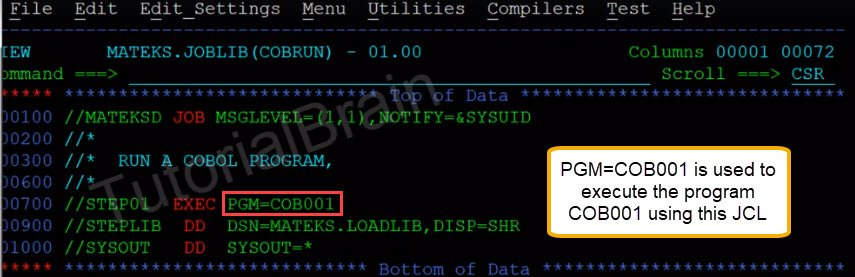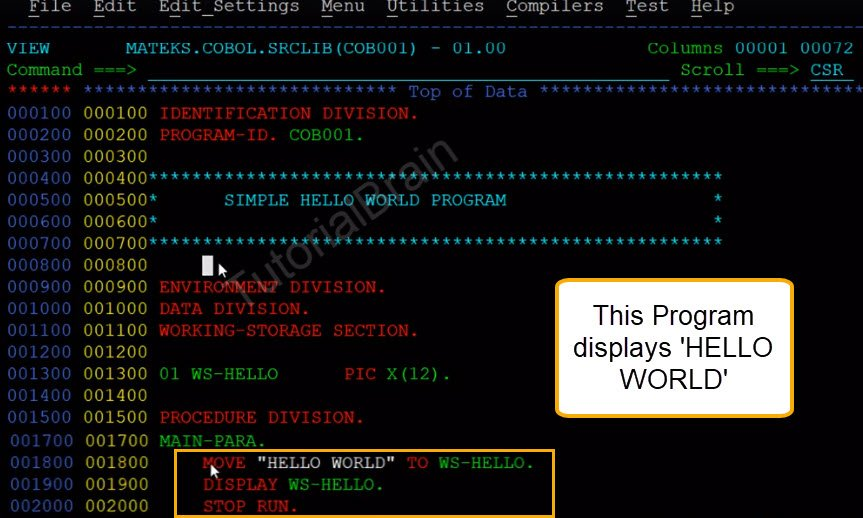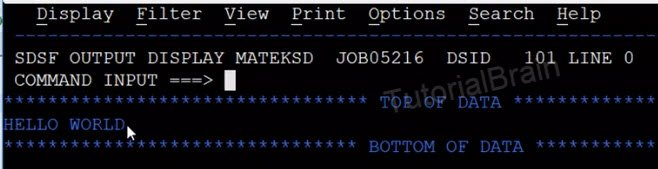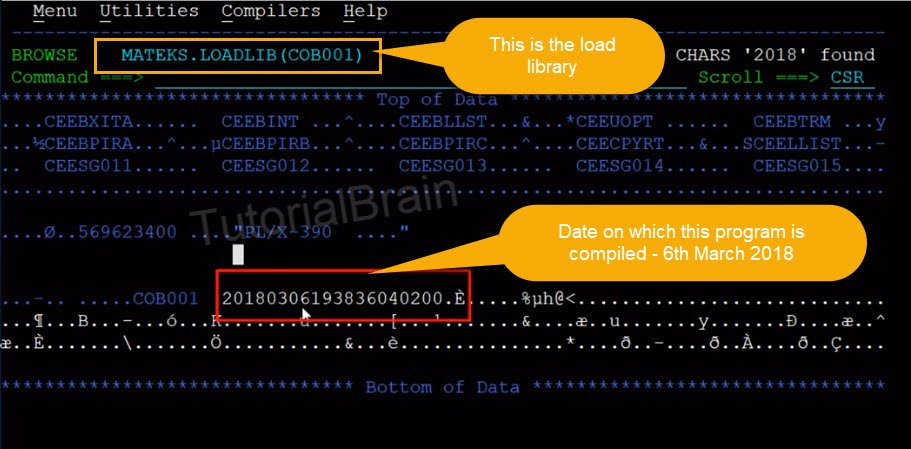# JCL PGM Parameter

## JCL PGM Parameter

JCL PGM parameter includes the program to be executed for that particular step.

Syntax:
PGM=PROGRAM-NAME

## Example to show JCL PGM Parameter

//STEP01   EXEC PGM=COB001You see that we have a step STEP01 and we have a program COB001 in this step. This program will be included using the PGM parameter.So, you can see that the program COB001 is a simple hello world program and this program just displays ‘Hello world’ in the output.

## Output

HELLO WORLDSo, if you want to run this program then you have to follow these steps –
1) You need to compile program COB001 using a compiled utility. This will generate the load module of the program which is also called as load library. The Load Library will look like this-2)You have to include this particular program using PGM Parameter and we have also done this process.
3)You have to include the load library from where this program needs to pick the load module.

## JCL Interview Question and Answers

1. If time mention on the jobcard statement is TIME=(,50) and if STEP1 uses the processor for 40 seconds, then the time allotted for step 2 will be

//STEP1 EXEC PGM=PGM1,TIME=(,35)
//STEP2 EXEC PGM=USES30,TIME=(,40)

a. 40 seconds
b. 5 seconds
c. 10 seconds
d. 15 seconds

d. 15 seconds

2. Consider the following code,

```//STEP1  EXEC  PGM=CINDY
//STEP2  EXEC  PGM=NEXT,COND=(4,EQ,STEP1)
//STEP3  EXEC  PGM=LAST,COND=((8,LT,STEP1),(0,LT,STEP2))
If STEP1 has returned CC=4
```
1. STEP2 will be bypassed and STEP3 will not be executed by assuming CC = 16 for STEP2
2. STEP2 will be bypassed and STEP3 will be executed by assuming CC = 0 for step2
3. STEP2 will be bypassed and STEP3 will be executed without performing return code test on STEP2
4. STEP2 will be executed and STEP3 will be executed without performing return code test on STEP2
1. STEP2 will be bypassed and STEP3 will be executed without performing return code test on STEP2